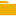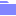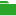# PQL Language

## Function LibrariesActionCommonDynamicGranularSemantic

## Pyramid Query Language Overview

The Pyramid Query Language or PQL (pronounced 'Prequel') is a language built into Pyramid's PYRANA engine to allow users to construct queries that can run against the many SQL data stacks that Pyramid can query natively. PQL includes a vast array of operations and functions that allows users to query data and build analytical logic.

### Unified Language

PQL is a unified language, that incorporates functions and methods for setting column aggregations, running analytical functions, as well as classic programmatic functions. To better understand the various tiers in PQL, it's best to compare it to other languages in the data space:

#### Querying Calculations

• GRANULAR: column aggregations and querying (usually done with languages like SQL). This type of language is used for querying relational databases, and often for aggregating column values. For example, sum all sales for each product in the transaction table.
• SEMANTIC: analytical methods, hierarchical logic, data point (or tuple) mathematics and advanced analytic queries (usually done with languages like MDX). This type of language is used for manipulating basic queries and aggregated results to produce higher level mathematical calculations. For instance, divide all sales figures for each state and show them as a percentage of their parent country.
• CONTEXT: analytical methods that extend the original semantic calculation model, and also include the reporting context in the equation (this is usually done in tools like Excel rather than a querying language). So, calculations can include 'context' logic that compares each row to the previous row in a query result, to find the rolling difference.

#### Computational Calculations

Beyond the three tiers of query-centric calculation lies three mathematical libraries of functions and methods:

• COMMON: This library includes classic mathematical and programmatic functions found in lower level, data agnostic languages (like Java, C# or VBA). These functions are used to perform logic on more basic data, without concern for data structures, context, or even source. For example, finding the absolute value of a number, or raising one number to the power of another.
• ACTION: This specialized library includes Pyramid-specific mathematical and programmatic functions like the Common library - earmarked for simplifying the logic needed to build formulas for triggers, dynamic text, and infographics, actions, dynamic URL web pages, inter-model interactions and jump buttons.

### The Blend

PQL blends all calculation functions and the common libraries into a single holistic engine, that can be used in the application as shown below.

The diagram shows:

• Granular methods can be created and used in Data Model, Formulate and Discovery context calculations.
• Granular calculations built in the context calculation and formulate tiers are really being executed in the data model tier
• Semantic methods can be created and used in Formulate and Discovery context calculations.
• Semantic calculations built in the context calculation tier are really being executed in the formulation tier
• Context calculations, by definition, can only be used in Discovery where report context exists.
• Common methods can be used in most places, including Data Flow calculated columns, Formulate formulas, context calculations, dynamic text logic in Illustrate, and triggers.
• Dynamic methods are available in all apps with triggers, dynamic text, and infographics
• Action methods are available in all the Discover and Present apps for actions, dynamic URLs, inter-model interactions

Note that Raw SQL methods relevant to each raw data source can be used in Custom SQL statements in Data Flow. These are unrelated to PQL.